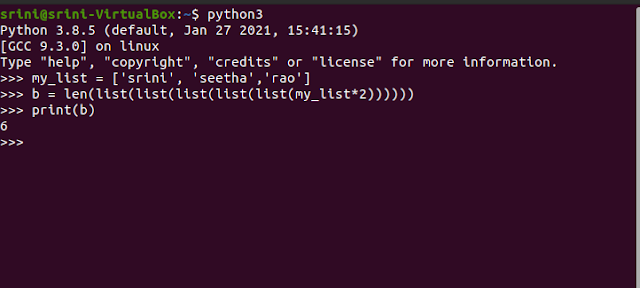# How to Use List Function in Python

Here's is an answer to calculate the length of the repeated lists. LIST is a principle data type in Python that holds a list of values. Usually strings.

## List() Function the Real Usage

You can do various operations with Lists. The best examples are REPEAT, APPEND, INSERT, EXTEND, COUNT. Still more you can do.

Note: This question is from a test recently held by an HR company. HR firms usually ask to take an online test to filter the job-seekers.1. Question
2. How to Find Length

Interview Question

The '* 2' says times the list is repeated. In this question, they have given '2' times.

my_list = ['srini', 'seetha', 'rao']
b = len(list(list(list(list(list(my_list*2))))))
Print (b)

1. 5
2. 6
3. 7
4. None

Explanation:

• In the first step, the '*' says, you repeated the List two times.
• It confuses the test-taker since they have given multiple functions of the list().

How to get Length

You can code the way I did. So that you can get the length correctly. So, the answer to the question is '6'. The List is repeated twice. There's no effect of multiple lists on the list.

Result (Ubuntu Linux OS)

At the command level, the given question entered. After pressing enter, it showed the result as '6'. That means the list (which has initially '3' elements') doubled. So the list length is now '6'.References